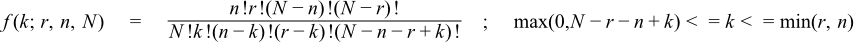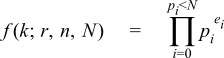#Boost C++ Libraries

...one of the most highly regarded and expertly designed C++ library projects in the world.

#### Hypergeometric Distribution

`#include <boost/math/distributions/hypergeometric.hpp>`
```namespace boost{ namespace math{

template <class RealType = double,
class Policy   = policies::policy<> >
class hypergeometric_distribution;

template <class RealType, class Policy>
class hypergeometric_distribution
{
public:
typedef RealType value_type;
typedef Policy   policy_type;
// Construct:
hypergeometric_distribution(unsigned r, unsigned n, unsigned N);
// Accessors:
unsigned total()const;
unsigned defective()const;
unsigned sample_count()const;
};

typedef hypergeometric_distribution<> hypergeometric;

}} // namespaces
```

The hypergeometric distribution describes the number of "events" k from a sample n drawn from a total population N without replacement.

Imagine we have a sample of N objects of which r are "defective" and N-r are "not defective" (the terms "success/failure" or "red/blue" are also used). If we sample n items without replacement then what is the probability that exactly k items in the sample are defective? The answer is given by the pdf of the hypergeometric distribution ```f(k; r, n, N)```, whilst the probability of k defectives or fewer is given by F(k; r, n, N), where F(k) is the CDF of the hypergeometric distribution.

NoteUnlike almost all of the other distributions in this library, the hypergeometric distribution is strictly discrete: it can not be extended to real valued arguments of its parameters or random variable.

The following graph shows how the distribution changes as the proportion of "defective" items changes, while keeping the population and sample sizes constant:Note that since the distribution is symmetrical in parameters n and r, if we change the sample size and keep the population and proportion "defective" the same then we obtain basically the same graphs:##### Member Functions
```hypergeometric_distribution(unsigned r, unsigned n, unsigned N);
```

Constructs a hypergeometric distribution with with a population of N objects, of which r are defective, and from which n are sampled.

```unsigned total()const;
```

Returns the total number of objects N.

```unsigned defective()const;
```

Returns the number of objects r in population N which are defective.

```unsigned sample_count()const;
```

Returns the number of objects n which are sampled from the population N.

##### Non-member Accessors

All the usual non-member accessor functions that are generic to all distributions are supported: Cumulative Distribution Function, Probability Density Function, Quantile, Hazard Function, Cumulative Hazard Function, mean, median, mode, variance, standard deviation, skewness, kurtosis, kurtosis_excess, range and support.

The domain of the random variable is the unsigned integers in the range [max(0, n + r - N), min(n, r)]. A domain_error is raised if the random variable is outside this range, or is not an integral value.

CautionThe quantile function will by default return an integer result that has been rounded outwards. That is to say lower quantiles (where the probability is less than 0.5) are rounded downward, and upper quantiles (where the probability is greater than 0.5) are rounded upwards. This behaviour ensures that if an X% quantile is requested, then at least the requested coverage will be present in the central region, and no more than the requested coverage will be present in the tails. This behaviour can be changed so that the quantile functions are rounded differently using Policies. It is strongly recommended that you read the tutorial Understanding Quantiles of Discrete Distributions before using the quantile function on the Hypergeometric distribution. The reference docs describe how to change the rounding policy for these distributions. However, note that the implementation method of the quantile function always returns an integral value, therefore attempting to use a Policy that requires (or produces) a real valued result will result in a compile time error.
##### Accuracy

For small N such that `N < boost::math::max_factorial<RealType>::value` then table based lookup of the results gives an accuracy to a few epsilon. `boost::math::max_factorial<RealType>::value` is 170 at double or long double precision.

For larger N such that `N < boost::math::prime(boost::math::max_prime)` then only basic arithmetic is required for the calculation and the accuracy is typically < 20 epsilon. This takes care of N up to 104729.

For ```N > boost::math::prime(boost::math::max_prime)``` then accuracy quickly degrades, with 5 or 6 decimal digits being lost for N = 110000.

In general for very large N, the user should expect to loose log10N decimal digits of precision during the calculation, with the results becoming meaningless for N >= 1015.

##### Testing

There are three sets of tests: our implementation is tested against a table of values produced by Mathematica's implementation of this distribution. We also sanity check our implementation against some spot values computed using the online calculator here http://stattrek.com/Tables/Hypergeometric.aspx. Finally we test accuracy against some high precision test data using this implementation and NTL::RR.

##### Implementation

The PDF can be calculated directly using the formula:However, this can only be used directly when the largest of the factorials is guaranteed not to overflow the floating point representation used. This formula is used directly when ```N < max_factorial<RealType>::value``` in which case table lookup of the factorials gives a rapid and accurate implementation method.

For larger N the method described in "An Accurate Computation of the Hypergeometric Distribution Function", Trong Wu, ACM Transactions on Mathematical Software, Vol. 19, No. 1, March 1993, Pages 33-43 is used. The method relies on the fact that there is an easy method for factorising a factorial into the product of prime numbers:Where pi is the i'th prime number, and ei is a small positive integer or zero, which can be calculated via:Further we can combine the factorials in the expression for the PDF to yield the PDF directly as the product of prime numbers:With this time the exponents ei being either positive, negative or zero. Indeed such a degree of cancellation occurs in the calculation of the ei that many are zero, and typically most have a magnitude or no more than 1 or 2.

Calculation of the product of the primes requires some care to prevent numerical overflow, we use a novel recursive method which splits the calculation into a series of sub-products, with a new sub-product started each time the next multiplication would cause either overflow or underflow. The sub-products are stored in a linked list on the program stack, and combined in an order that will guarantee no overflow or unnecessary-underflow once the last sub-product has been calculated.

This method can be used as long as N is smaller than the largest prime number we have stored in our table of primes (currently 104729). The method is relatively slow (calculating the exponents requires the most time), but requires only a small number of arithmetic operations to calculate the result (indeed there is no shorter method involving only basic arithmetic once the exponents have been found), the method is therefore much more accurate than the alternatives.

For much larger N, we can calculate the PDF from the factorials using either lgamma, or by directly combining lanczos approximations to avoid calculating via logarithms. We use the latter method, as it is usually 1 or 2 decimal digits more accurate than computing via logarithms with lgamma. However, in this area where N > 104729, the user should expect to loose around log10N decimal digits during the calculation in the worst case.

The CDF and its complement is calculated by directly summing the PDF's. We start by deciding whether the CDF, or its complement, is likely to be the smaller of the two and then calculate the PDF at k (or k+1 if we're calculating the complement) and calculate successive PDF values via the recurrence relations:Until we either reach the end of the distributions domain, or the next PDF value to be summed would be too small to affect the result.

The quantile is calculated in a similar manner to the CDF: we first guess which end of the distribution we're nearer to, and then sum PDFs starting from the end of the distribution this time, until we have some value k that gives the required CDF.

The median is simply the quantile at 0.5, and the remaining properties are calculated via:Copyright © 2006-2010, 2012, 2013 Paul A. Bristow, Christopher Kormanyos, Hubert Holin, Bruno Lalande, John Maddock, Johan Råde, Gautam Sewani, Benjamin Sobotta, Thijs van den Berg, Daryle Walker and Xiaogang Zhang Distributed under the Boost Software License, Version 1.0. (See accompanying file LICENSE_1_0.txt or copy at http://www.boost.org/LICENSE_1_0.txt)